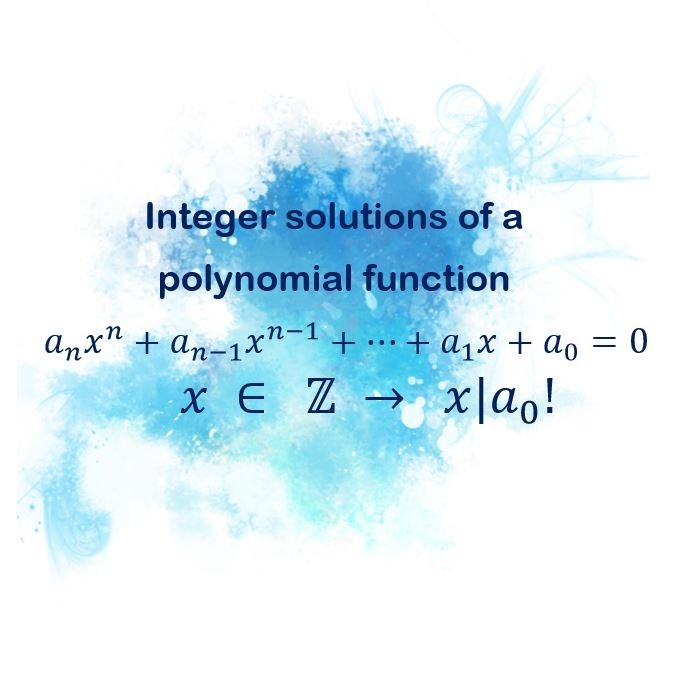# Finding an integer solutions of a polynomial function

Integer solutions of a polynomial function theorem says: If a polynomial function $a_n x^n + a_{n – 1} x^{n – 1} + … + a_1 x + a_0 = 0$ with integer coefficients has an integer solution, $a \not= 0$, then that solution is the divisor of free coefficient $a_0$ .

As an addition to this theorem, for every whole number k, number $\alpha – k$ is a divisor of $\ f(k)$.Example 1. Find all integer roots of $x^3 – 8x^2 + 25x – 26 = 0$.

$f(x) = x^3 – 8x^2 + 25x – 26$

The set of dividers is, according to the last theorem, the set of dividers of number -26.

$a \in \{-1, 1, -2, 2, -13, 13, -26, 26 \}$

It would take a lot of time to check all these solutions so we’ll first eliminate few of them.

We can take any whole number k, for example here we’ll take k = 1. According to the addition to the theorem, for every integer root of this polynomial -$\alpha$ , polynomial $\alpha – 1$ has to divide f(k) without the remainder.

Now let’s take a look at a set of solutions for $\alpha – 1$.

$\alpha – 1 \in \{-2, 0, -3, 1, -14, 12, -27, 25 \}$

$f(1) = 1^3 – 8 * 1^2 + 25 * 1 – 26$

$f(1) = -8$

Now we can eliminate every with which – 8 is not divisible.

$\alpha – 1 \in$ {-2, 0, -3, 1, -14, 12, -27, 25}

$\alpha \in \{-1, 2 \}$

Now we have significantly smaller set of possible solutions. Now we have to check both of them to see are they roots of given equation.This means that number 2 is the only whole root of this equation.